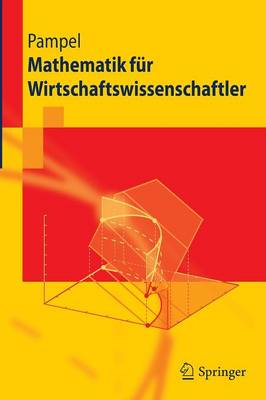•# Mathematik Fur Wirtschaftswissenschaftler - Springer-Lehrbuch (Paperback)

(author)
£25.99
Paperback 318 Pages / Published: 11/06/2009
• We can order this

Usually dispatched within 3 weeks

Ob Betriebs- oder Volkswirtschaftslehre, in den Wirtschaftswissenschaften wird mehr Mathematik verwendet, als viele Studierende erwarten. Schon in den ersten Semestern werden mathematische Methoden genutzt, z. B. um Marktgleichgewichte zu bestimmen. Das Lehrbuch behandelt mathematisches Basiswissen und einige Erweiterungen wie etwa Eigenwerte und Eigenvektoren. Dabei erlautert jedes Kapitel oekonomische Fragestellungen, zu deren Loesung der jeweilige Stoff des Abschnitts Anwendung findet. Mit farbigen Abbildungen und kompakter Formelsammlung.

Publisher: Springer-Verlag Berlin and Heidelberg GmbH & Co. KG
ISBN: 9783642044892
Number of pages: 318
Weight: 463 g
Dimensions: 234 x 156 x 18 mm
Edition: 2010 ed.

MEDIA REVIEWS

From the reviews: The textbook can be used as an introduction into the field of mathematics for business economists as well as for economists. It is written for undergraduates . presents a large number of graphical illustrations as well as practical solutions which enable the reader to understand the mathematical topics quite easily. The book contains a collection of formulas in the appendix as well as a short overview of higher level mathematical textbooks and exercises. (Herbert S. Buscher, Zentralblatt MATH, Vol. 1184, 2010)"

From the reviews:

The textbook can be used as an introduction into the field of mathematics for business economists as well as for economists. It is written for undergraduates . presents a large number of graphical illustrations as well as practical solutions which enable the reader to understand the mathematical topics quite easily. The book contains a collection of formulas in the appendix as well as a short overview of higher level mathematical textbooks and exercises. (Herbert S. Buscher, Zentralblatt MATH, Vol. 1184, 2010)"

From the reviews:

"The textbook can be used as an introduction into the field of mathematics for business economists as well as for economists. It is written for undergraduates ... . presents a large number of graphical illustrations as well as practical solutions which enable the reader to understand the mathematical topics quite easily. The book contains a collection of formulas in the appendix as well as a short overview of higher level mathematical textbooks and exercises." (Herbert S. Buscher, Zentralblatt MATH, Vol. 1184, 2010)